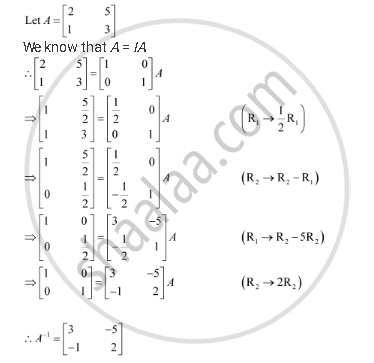Share

# Find the Inverse of Each of the Matrices, If It Exists. [(2,5),(1,3)] - CBSE (Science) Class 12 - Mathematics

#### Question

Find the inverse of each of the matrices, if it exists.

[(2,5),(1,3)]

#### SolutionIs there an error in this question or solution?

#### Video TutorialsVIEW ALL 

Solution Find the Inverse of Each of the Matrices, If It Exists. [(2,5),(1,3)] Concept: Invertible Matrices.
S